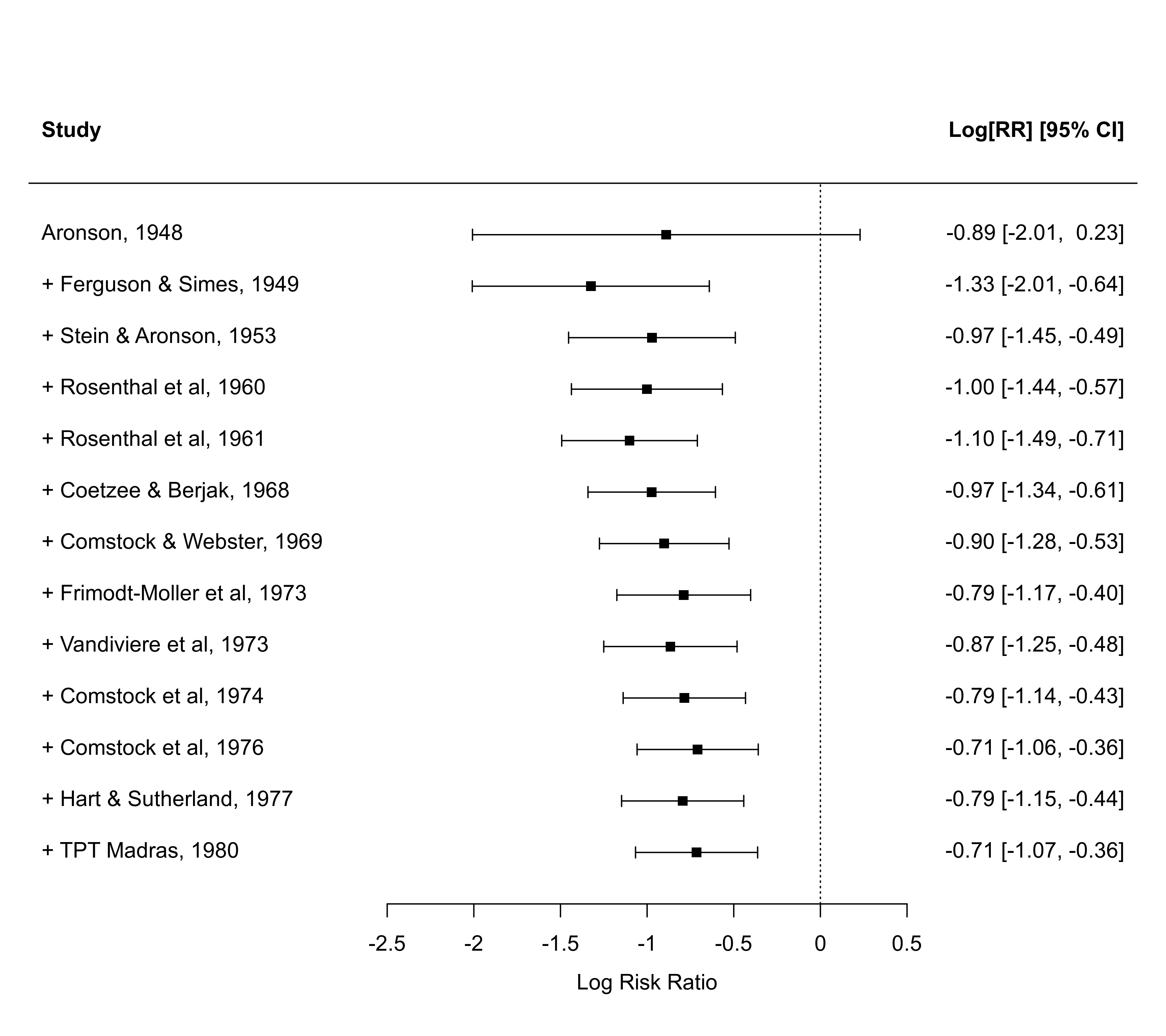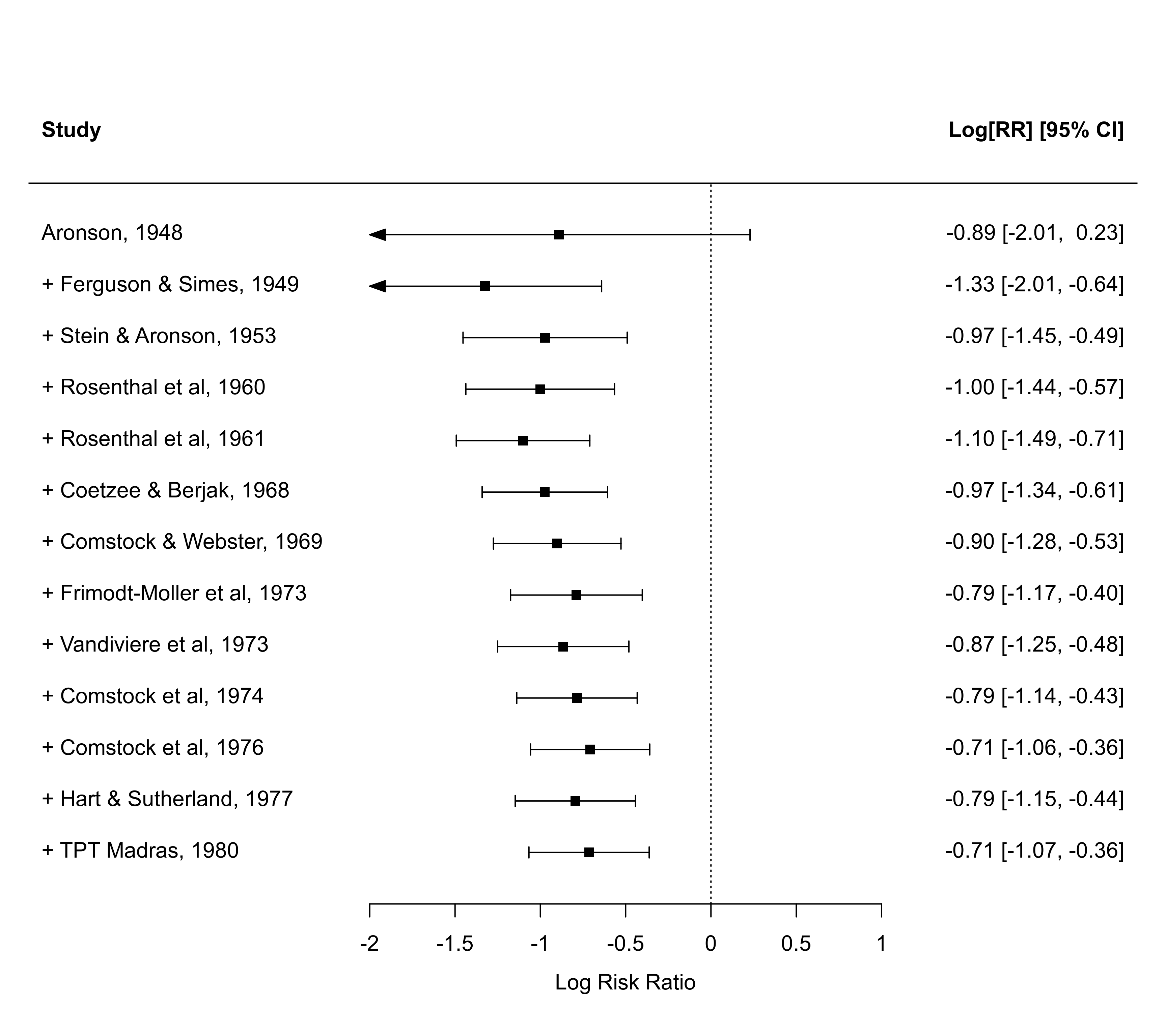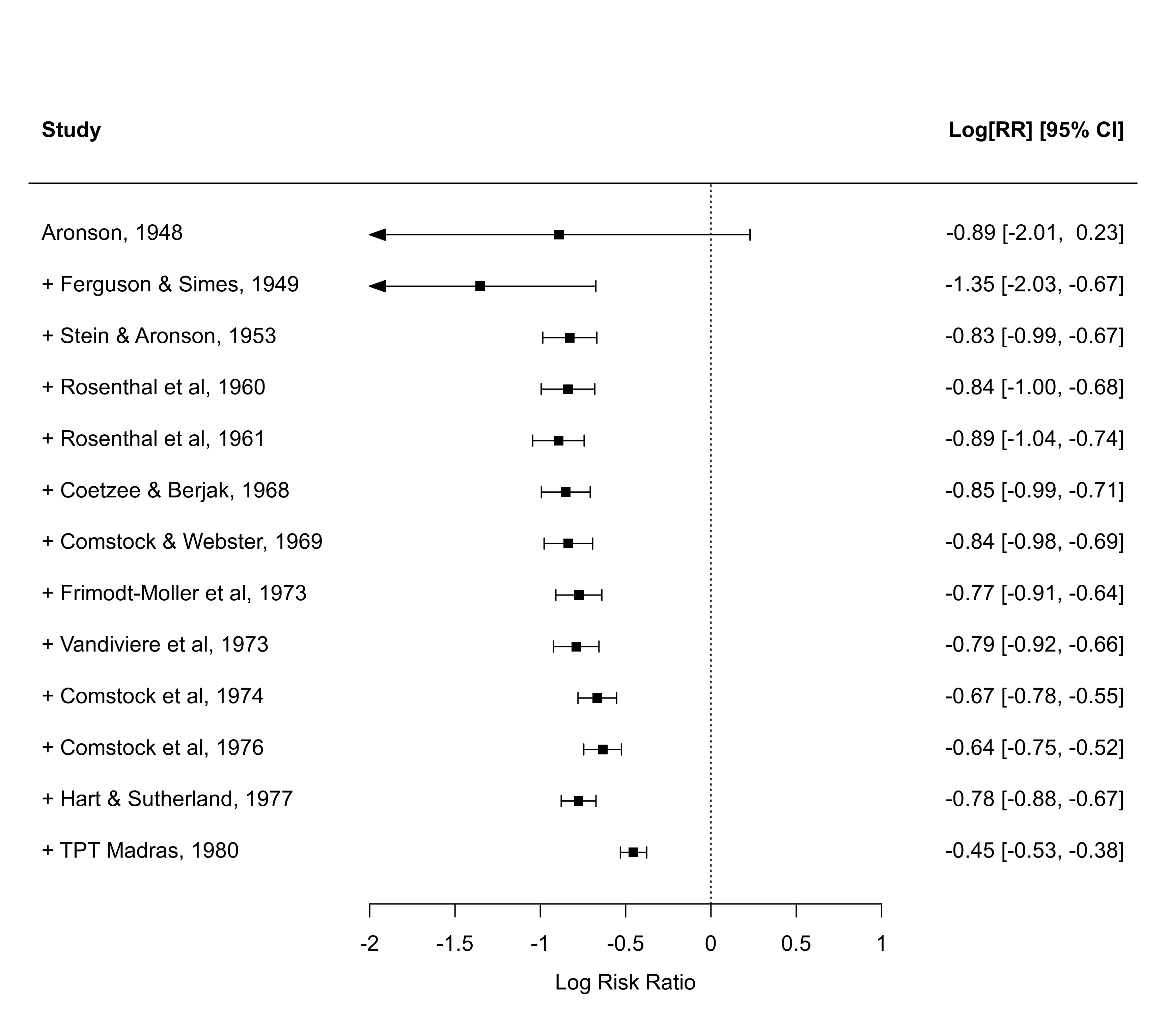Function to create forest plots for objects of class "cumul.rma".

# S3 method for cumul.rma
xlim, alim, olim, ylim, at, steps=5,
level=x\$level, refline=0, digits=2L, width,
xlab, ilab, ilab.xpos, ilab.pos,
transf, atransf, targs, rows,
efac=1, pch, psize, col,
lty, fonts, cex, cex.lab, cex.axis, ...)

## Arguments

x

an object of class "cumul.rma" obtained with cumul.

annotate

logical to specify whether annotations should be added to the plot (the default is TRUE).

logical to specify whether column headings should be added to the plot (the default is FALSE). Can also be a character vector to specify the left and right headings (or only the left one).

xlim

horizontal limits of the plot region. If unspecified, the function tries to set the horizontal plot limits to some sensible values.

alim

the x-axis limits. If unspecified, the function tries to set the x-axis limits to some sensible values.

olim

optional argument to specify observation/outcome limits. If unspecified, no limits are used.

ylim

the y-axis limits of the plot. If unspecified, the function tries to set the y-axis limits to some sensible values.

at

position of the x-axis tick marks and corresponding labels. If unspecified, the function tries to set the tick mark positions/labels to some sensible values.

steps

the number of tick marks for the x-axis (the default is 5). Ignored when the positions are specified via the at argument.

level

numeric value between 0 and 100 to specify the confidence interval level (the default is to take the value from the object).

refline

numeric value to specify the location of the vertical ‘reference’ line (the default is 0). The line can be suppressed by setting this argument to NA.

digits

integer to specify the number of decimal places to which the tick mark labels of the x-axis and the annotations should be rounded (the default is 2L). Can also be a vector of two integers, the first to specify the number of decimal places for the annotations, the second for the x-axis labels. When specifying an integer (e.g., 2L), trailing zeros after the decimal mark are dropped for the x-axis labels. When specifying a numeric value (e.g., 2), trailing zeros are retained.

width

optional integer to manually adjust the width of the columns for the annotations (either a single integer or a vector of the same length as the number of annotation columns).

xlab

title for the x-axis. If unspecified, the function tries to set an appropriate axis title.

ilab

optional vector, matrix, or data frame providing additional information about the studies that should be added to the plot.

ilab.xpos

numeric vector to specify the horizontal position(s) of the variable(s) given via ilab (must be specified if ilab is specified).

ilab.pos

integer(s) (either 1, 2, 3, or 4) to specify the alignment of the vector(s) given via ilab (2 means right, 4 mean left aligned). If unspecified, the default is to center the labels.

transf

optional argument to specify a function to transform the estimates and confidence interval bounds (e.g., transf=exp; see also transf). If unspecified, no transformation is used.

atransf

optional argument to specify a function to transform the x-axis labels and annotations (e.g., atransf=exp; see also transf). If unspecified, no transformation is used.

targs

optional arguments needed by the function specified via transf or atransf.

rows

optional vector to specify the rows (or more generally, the horizontal positions) for plotting the outcomes. Can also be a single value to specify the row (horizontal position) of the first outcome (the remaining outcomes are then plotted below this starting row). If unspecified, the function sets this value automatically.

efac

vertical expansion factor for confidence interval limits and arrows. The default value of 1 should usually work okay. Can also be a vector of two numbers, the first for CI limits, the second for arrows.

pch

plotting symbol to use for the estimates. By default, a filled square is used. See points for other options. Can also be a vector of values.

psize

numeric value to specify the point sizes for the estimates (the default is 1). Can also be a vector of values.

col

optional character string to specify the color to use for plotting ("black" is used by default if not specified). Can also be a vector.

lty

optional character string to specify the line type for the confidence intervals. If unspecified, the function sets this to "solid" by default.

fonts

optional character string to specify the font to use for the study labels, annotations, and the extra information (if specified via ilab). If unspecified, the default font is used.

cex

optional character and symbol expansion factor. If unspecified, the function tries to set this to a sensible value.

cex.lab

optional expansion factor for the x-axis title. If unspecified, the function tries to set this to a sensible value.

cex.axis

optional expansion factor for the x-axis labels. If unspecified, the function tries to set this to a sensible value.

...

other arguments.

## Details

The plot shows the estimated (average) outcome with corresponding confidence interval as one study at a time is added to the analysis.

## Note

The function tries to set some sensible values for the optional arguments, but it may be necessary to adjust these in certain circumstances.

The function actually returns some information about the chosen values invisibly. Printing this information is useful as a starting point to make adjustments to the plot.

If the number of studies is quite large, the labels, annotations, and symbols may become quite small and impossible to read. Stretching the plot window vertically may then provide a more readable figure (one should call the function again after adjusting the window size, so that the label/symbol sizes can be properly adjusted). Also, the cex, cex.lab, and cex.axis arguments are then useful to adjust the symbol and text sizes.

If the horizontal plot and/or x-axis limits are set manually, then the horizontal plot limits (xlim) must be at least as wide as the x-axis limits (alim). This restriction is enforced inside the function.

If the outcome measure used for creating the plot is bounded (e.g., correlations are bounded between -1 and +1, proportions are bounded between 0 and 1), one can use the olim argument to enforce those limits (the observed outcomes and confidence intervals cannot exceed those bounds then).

The lty argument can also be a vector of two elements, the first for specifying the line type of the individual CIs ("solid" by default), the second for the line type of the horizontal line that is automatically added to the plot ("solid" by default; set to "blank" to remove it).

## Author

Wolfgang Viechtbauer wvb@metafor-project.org https://www.metafor-project.org

forest for an overview of the various forest functions.

cumul for the function to create cumul.rma objects.

## Examples

### calculate log risk ratios and corresponding sampling variances
dat <- escalc(measure="RR", ai=tpos, bi=tneg, ci=cpos, di=cneg, data=dat.bcg)

### fit random-effects model
res <- rma(yi, vi, data=dat, slab=paste(author, year, sep=", "))

### draw cumulative forest plots
x <- cumul(res, order=year)